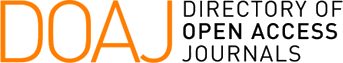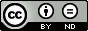### Concordance-based Kendall's Correlation for Computationally-Light vs. Computationally-Heavy Centrality Metrics: Lower Bound for Correlation

Natarajan Meghanathan

#### Abstract

We identify three different levels of correlation (pair-wise relative ordering, network-wide ranking and linear regression) that could be assessed between a computationally-light centrality metric and a computationally-heavy centrality metric for real-world networks. The Kendall's concordance-based correlation measure could be used to quantitatively assess how well we could consider the relative ordering of two vertices vi and vj with respect to a computationally-light centrality metric as the relative ordering of the same two vertices with respect to a computationally-heavy centrality metric. We hypothesize that the pair-wise relative ordering (concordance)-based assessment of the correlation between centrality metrics is the most strictest of all the three levels of correlation and claim that the Kendall's concordance-based correlation coefficient will be lower than the correlation coefficient observed with the more relaxed levels of correlation measures (linear regression-based Pearson's product-moment correlation coefficient and the network wide ranking-based Spearman's correlation coefficient). We validate our hypothesis by evaluating the three correlation coefficients between two sets of centrality metrics: the computationally-light degree and local clustering coefficient complement-based degree centrality metrics and the computationally-heavy eigenvector centrality, betweenness centrality and closeness centrality metrics for a diverse collection of 50 real-world networks.

#### Keywords

relative ordering, ranking, linear regression, centrality, correlation

#### Full Text:

PDF

DOI: https://doi.org/10.20532/cit.2017.1003492This work is licensed under a Creative Commons Attribution-NoDerivatives 4.0 International License.